# SAT II Chemistry : Compounds and Molecules

## Example Questions

### Example Question #1 : Sat Subject Test In Chemistry

Based on VSEPR theory and electron configurations, which of the following molecules is not matched with its correct geometry?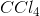- Tetrahedral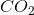- Linear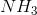- Trigonal planar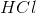- Linear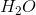- Bent (v-shaped)- Trigonal planar

Explanation:has three bonding pairs and one non-bonding pair, which is the geometry for trigonal pyramidal, not trigonal planar.

### Example Question #1 : Compounds And Molecules

What is the molecular geometry of?

Trigonal pyramidal

Octahedral

Tetrahedral

Square planar

Square pyramidal

Square pyramidal

Explanation:

There are six electron domains inhas five bonding and one nonbonding pairs of electrons. The electron domain geometry ofis octahedral. When a molecule has octahedral electron domain geometry and has five bonding and one nonbonding pairs, the molecular geometry is square pyramidal.

### Example Question #1 : Naming Compounds

Which of the following is the correct name of?

Dinitrogen pentoxide

Nitrogen monoxide

Dinitrogen tetraoxide

Nitrogen dioxide

Dinitrogen monoxide

Dinitrogen tetraoxide

Explanation:

In order to answer the question, it is important to first determine what kind of bonding is occurring in order to properly name the molecule. When nitrogen and oxygen form anmolecule, electrons are shared between the atoms, so a covalent bond is formed.

Because a covalent bond is formed, we can determine that name of the molecule will be based on number of each atom in the molecule. There are two nitrogen atoms in the resulting molecule, so the prefix in front of the "nitrogen" part of the name will be "di-," meaning two. There are four oxygen atoms in the resulting molecule, so the prefix in front of the "oxygen" part of the name will be "tetra," meaning four.

The molecules names will be arranged alphabetically, and the second atom will have "-ide" as the suffix. Therefore, the correct answer is "dinitrogen tetroxide."

### Example Question #1 : Complex And Polyatomic Ions

What is the formula for the compound formed from calcium and sulfate ions?Explanation:

In order to answer this question correctly, it is important to have knowledge of the formation of ionic bonds, polyatomic ions, and the periodic table. First, it is important to notice that calcium ions and sulfate ions will form an ionic bond. Knowing this, it can be understood that valance electrons will be exchanged between the ions and therefore, oppositely charged ions that are attracted to each other will be formed.

Calcium is in the second column of the periodic table, so it will provide two ions to the sulfate polyatomic ion. This will form a calcium cation () and a sulfate polyatomic anion ().

One calcium ion provides two electrons, which are both accepted by a sulfate polyatomic ion, so the ratio of calcium ions used to sulfate polyatomic ions used is 1:1. This means that only one of each ion is needed to form the compound, making the formula.

Initially it may be tempting to write the formula as; however, since both ions have a "2" subscript in this form, it is redundant to the formula in this way. Only one of each ion is needed to form the compound.

### Example Question #1 : Compounds

All of the following are properties of zinc. Which of the following are chemical properties of zinc?

A. Zinc produces hydrogen gas when placed in acid.

B. Zinc melts at.

C. The density of zinc is.

D. Zinc is a grey metal.

E. Zinc corrodes in moist air.

B

A, C, and E

A and E

B and C

C

A and E

Explanation:

Physical properties are aspects of matter that can be observed and measured without changing it. Chemical properties measure the potential for undergoing a chemical change. How zinc reacts to acid and in moist air are both chemical properties because the zinc is undergoing chemical change in each environment. The color, density, and melting point of the zinc can all be observed without changing the nature of the matter, so these are physical properties of zinc.

### Example Question #1 : Compounds

Which of the following are strong electrolytes?Two

Three

Four

One

All five

Three

Explanation:

Strong electrolytes are substances that exists in solutions as only ions. These substances dissociate 100% in solutions. Strong acids, strong bases, and salts are all examples of strong electrolytes.is a strong acid.is a salt.is a salt.is a weak acid.is a weak base. Therefore the answer is three.

### Example Question #2 : Compounds And Molecules

How many of the following compounds are ionic?Two

All five

Three

Zero

One

Two

Explanation:

Ionic compounds are chemical compounds made of charged ions held together by forces called ionic bonding. Ionic bonds are formed by transfers of valance electrons, which create charged atoms (ions) which are attracted to each other because they have opposite charges. This is not be confused with covalent bonding, in which atoms form an attraction by sharing electrons.contains a positively chargedion and negatively chargedion which form an ionic bond.contains two positively chargedions and a negatively chargedion which form an ionic bond.,, andare all examples of molecules that contain covalent bonds. None of them contains any charged ions. The answer is therefore "two," referring to theandmolecules.

### Example Question #1 : Bonding And Forces

Which of the following does not exhibit hydrogen bonding as an intermolecular force?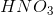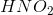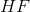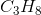Explanation:

Hydrogen bonding occurs between hydrogen and a very electronegative atom, which is usually fluorine, oxygen, or nitrogen. Thus, hydrogen bonding does not occur in.

### Example Question #1 : Compounds And Molecules

In a solution, a weak electrolyte exists predominately as which of the following?

Ions

Molecules

Isotopes

Polyatomic ions

Electrons